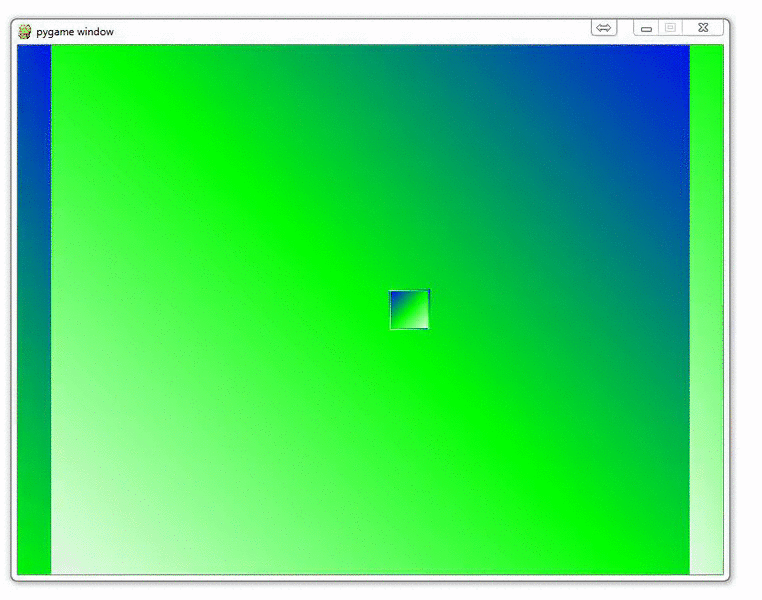## Adding a ground in OpenGL

While it is not the immediate goal for this project, sometimes you may want to create a floor, ground, ceiling, or wall in your OpenGL game with Python. Here, I will show you how you might go about doing that.

If you recall how we made our cubes, we specified the four vertices that we wanted to color in, and that was it. Creating a wall of some sort is done the very same way. Just specify the vertices.

So, to begin, we'll need to know what makes up the surface, where those vertices are, and then actually draw them with OpenGL.

First:

```ground_surfaces = (0,1,2,3)
```

Not totally necessary here, since we could just create a for loop with range, but I figured I should keep the same structure as before to not confuse anyone. So, 0,1,2, and 3 are the vertices that will make up our ground. We're using those vertices from a ground_vertices tuple:

```ground_vertices = (
(-10,-0.1,50),
(10,-0.1,50),
(-10,-0.1,-300),
(10,-0.1,-300),

)
```

Finally, we're ready to actually create the ground:

```def Ground():

x = 0
for vertex in ground_vertices:
x+=1
glColor3fv((0,1,1))
glVertex3fv(vertex)

glEnd()
```

Easy enough! Now, as with any graphics rendering, keep in mind the order that you draw things. You should probably draw the ground last. If you had a character, then you'd probably draw the ground, then the character last. Why is this?

If you draw the ground first, then draw the cubes, imagine there is a cube beyond the "wall." In that case, that cube that should be "beyond" the wall will still appear, just look small or something, it will immediately disorient the user, and look just plain wrong, because it is!

I've also gone ahead and placed all of the cubes on that "ground." This version of the game is actually quite close to our original 2D game. You could take this one further. This one will use far less processing than our eventual version, and may be a better theory for web-based games, or phone applications.

Here's my full version of the code (note the changes about where cubes are rendered so they are rendered on the ground):

```import pygame
from pygame.locals import *
from OpenGL.GL import *
from OpenGL.GLU import *
import random

vertices = (
(1, -1, -1),
(1, 1, -1),
(-1, 1, -1),
(-1, -1, -1),
(1, -1, 1),
(1, 1, 1),
(-1, -1, 1),
(-1, 1, 1)
)

edges = (
(0,1),
(0,3),
(0,4),
(2,1),
(2,3),
(2,7),
(6,3),
(6,4),
(6,7),
(5,1),
(5,4),
(5,7)
)

surfaces = (
(0,1,2,3),
(3,2,7,6),
(6,7,5,4),
(4,5,1,0),
(1,5,7,2),
(4,0,3,6)
)

colors = (
(1,0,0),
(0,1,0),
(0,0,1),
(0,1,0),
(1,1,1),
(0,1,1),
(1,0,0),
(0,1,0),
(0,0,1),
(1,0,0),
(1,1,1),
(0,1,1),
)

ground_surfaces = (0,1,2,3)

ground_vertices = (
(-10,-0.1,50),
(10,-0.1,50),
(-10,-0.1,-300),
(10,-0.1,-300),

)

def Ground():

x = 0
for vertex in ground_vertices:
x+=1
glColor3fv((0,1,1))
glVertex3fv(vertex)

glEnd()

def Cube():

for surface in surfaces:

for vertex in surface:
x+=1
glColor3fv(colors[x])
glVertex3fv(vertices[vertex])

glEnd()

glBegin(GL_LINES)
for edge in edges:
for vertex in edge:
glVertex3fv(vertices[vertex])
glEnd()

def set_vertices(max_distance):
x_value_change = random.randrange(-5,5)
y_value_change = 0#random.randrange(-10,10)
z_value_change = random.randrange(-1*max_distance,-20)

new_vertices = []
for vert in vertices:
new_vert = []

new_x= vert + x_value_change
new_y= vert + y_value_change
new_z= vert + z_value_change

new_vert.append(new_x)
new_vert.append(new_y)
new_vert.append(new_z)

new_vertices.append(new_vert)

return new_vertices

def Cubes(new_vertices):

for surface in surfaces:
x = 0

for vertex in surface:
x+=1
glColor3fv(colors[x])
glVertex3fv(new_vertices[vertex])

glEnd()

# CUT LINES BC THEY HURT PROCESSING
##    glBegin(GL_LINES)
##    for edge in edges:
##        for vertex in edge:
##            glVertex3fv(new_vertices[vertex])
##    glEnd()

def main():
pygame.init()
display = (800,600)
pygame.display.set_mode(display, DOUBLEBUF|OPENGL)

gluPerspective(45, (display/display), 0.1, 150.0)
glTranslatef(random.randrange(-5,5),0, -20)

x_move = 0
y_move = 0

max_distance = 300

cube_dict = {}

for x in range(60):
cube_dict[x] = set_vertices(max_distance)

object_passed = False

while not object_passed:
for event in pygame.event.get():
if event.type == pygame.QUIT:
pygame.quit()
quit()

if event.type == pygame.KEYDOWN:

if event.key == pygame.K_LEFT:
x_move = 0.3

if event.key == pygame.K_RIGHT:
x_move = -0.3

if event.key == pygame.K_UP:
y_move = -0.3

if event.key == pygame.K_DOWN:
y_move = 0.3

if event.type == pygame.KEYUP:
if event.key == pygame.K_LEFT:
x_move = 0

if event.key == pygame.K_RIGHT:
x_move = 0

if event.key == pygame.K_UP:
y_move = 0

if event.key == pygame.K_DOWN:
y_move = 0

x = glGetDoublev(GL_MODELVIEW_MATRIX)

camera_x = x
camera_y = x
camera_z = x

glTranslatef(x_move,y_move,3)

glClear(GL_COLOR_BUFFER_BIT|GL_DEPTH_BUFFER_BIT)
Ground()
for each_cube in cube_dict:
Cubes(cube_dict[each_cube])

pygame.display.flip()

main()

```

The next tutorial:• OpenGL with PyOpenGL introduction and creation of Rotating Cube

• Coloring Surfaces as well as understand some of the basic OpenGL code

• Understanding navigation within the 3D environment via OpenGL

• Moving the player automatically towards the cube

• Random Cube Position

• Adding Many Cubes to our Game

• Adding a ground in OpenGL
• Infinite flying cubes

• Optimizing the processing for infinite cubes